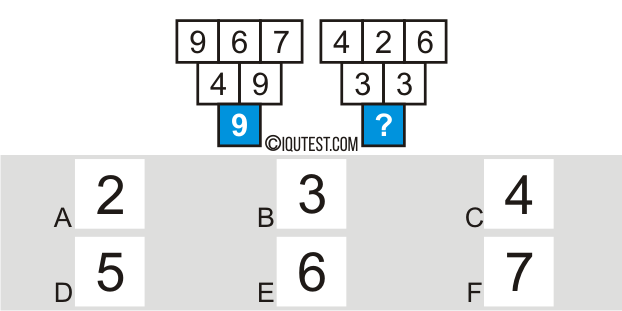# IQ Test Numerical [Number Nets]

Improve the ability of logic, mathematical problem solving using mathematical approach by exercising 20 questions of numerical test below.

Figure it out what the right number of answers by looking at the combination patterns of rows of numbers and rows of shapes, which are interconnected with each other. Choose one of the right answers according to the existing pattern. See the example of numerical number test below.

Example Question:
Find the missing number!Explanation: On the right box first row: (6 + 2 ) – 4 = 4

second row: (3 + 3) – 4 = 2

Numerical Test Number Nets
• 20 questions
• Numerical, Logical, Mathematical
• Time: 20 minutes

Challenge Your IQ with Engaging Brain GamesIQuTest.com Would you like to receive notifications on latest updates? No Yes